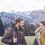# Proof Contest Day 4

In the triangle $ABC$ the point $J$ is the center of the excircle opposite to $A$. This excircle is tangent to the side $BC$ at $M$, and to the lines $AB$ and $AC$ at $K$ and $L$ respectively. The lines $LM$ and $BJ$ meet at $F$, and the lines $KM$ and $CJ$ meet at $G$. Let $S$ be the point of intersection of the lines $AF$ and $BC$, and let $T$ be the point of intersection of the lines $AG$ and $BC$. Prove that $M$ is the midpoint of $ST$.

Not originalNote by Department 8
5 years, 5 months ago

This discussion board is a place to discuss our Daily Challenges and the math and science related to those challenges. Explanations are more than just a solution — they should explain the steps and thinking strategies that you used to obtain the solution. Comments should further the discussion of math and science.

When posting on Brilliant:

• Use the emojis to react to an explanation, whether you're congratulating a job well done , or just really confused .
• Ask specific questions about the challenge or the steps in somebody's explanation. Well-posed questions can add a lot to the discussion, but posting "I don't understand!" doesn't help anyone.
• Try to contribute something new to the discussion, whether it is an extension, generalization or other idea related to the challenge.
• Stay on topic — we're all here to learn more about math and science, not to hear about your favorite get-rich-quick scheme or current world events.

MarkdownAppears as
*italics* or _italics_ italics
**bold** or __bold__ bold
- bulleted- list
• bulleted
• list
1. numbered2. list
1. numbered
2. list
Note: you must add a full line of space before and after lists for them to show up correctly
paragraph 1paragraph 2

paragraph 1

paragraph 2

[example link](https://brilliant.org)example link
> This is a quote
This is a quote
    # I indented these lines
# 4 spaces, and now they show
# up as a code block.

print "hello world"
# I indented these lines
# 4 spaces, and now they show
# up as a code block.

print "hello world"
MathAppears as
Remember to wrap math in $$ ... $$ or $ ... $ to ensure proper formatting.
2 \times 3 $2 \times 3$
2^{34} $2^{34}$
a_{i-1} $a_{i-1}$
\frac{2}{3} $\frac{2}{3}$
\sqrt{2} $\sqrt{2}$
\sum_{i=1}^3 $\sum_{i=1}^3$
\sin \theta $\sin \theta$
\boxed{123} $\boxed{123}$

## Comments

Sort by:

Top Newest

This is 2012 IMO Problem 1. No chance that I can solve it :P

- 5 years, 5 months ago

Log in to reply

Upload the solution

- 5 years, 5 months ago

Log in to reply

I did not get what you mean. I have not solved the problem. Just copy and paste this problem in google and you will find the solutions when you click the Aops if you were asking me for that.

- 5 years, 5 months ago

Log in to reply

Hint: There are cyclic shapes in the diagram. Prove that $J$ is the circumcenter of $AST$

- 5 years, 5 months ago

Log in to reply

@Xuming Liang, gave a nice hint but here is the official solution

- 5 years, 5 months ago

Log in to reply

More solutions can be found here

- 5 years, 5 months ago

Log in to reply

×

Problem Loading...

Note Loading...

Set Loading...